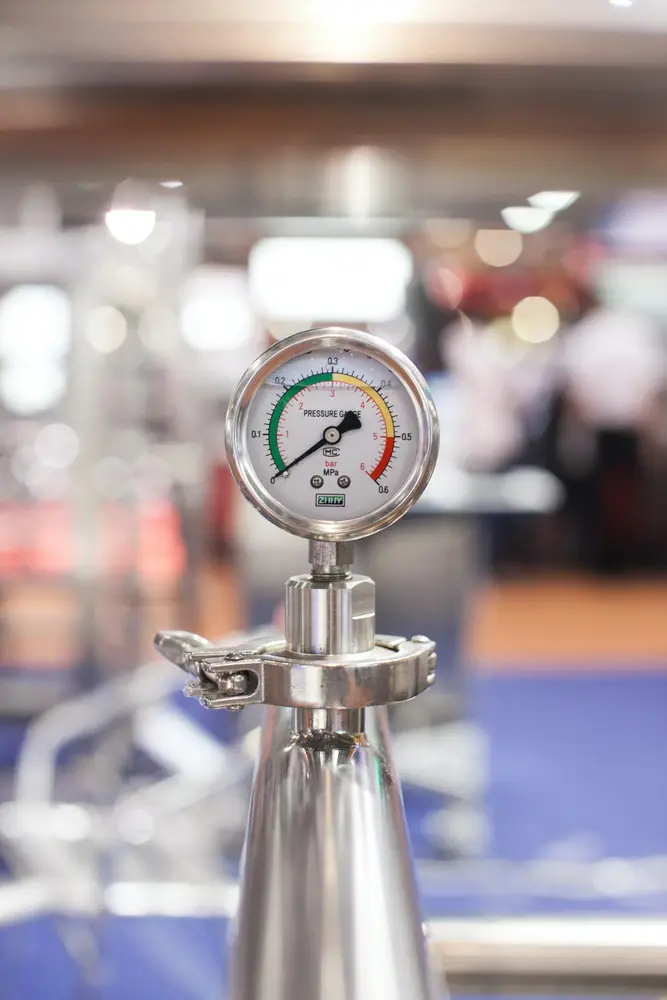# Forces

• Forces are usually a push or a pull.
• Forces are measured using a newtonmeter and the unit of force is Newtons.
• Forces work in pairs - as you push down on a table the table pushes up with equal force.
• When there is no change in velocity or shape of an object then the forces are balanced e.g. book on table
• When there is a change in velocity or shape of an object then the forces are not balanced e.g. person falling through ice.
• An example of a force is gravitational force. To work out the weight of an object (with gravity working on it) you use the following formula:
• Weight (N) = mass (kg) x gravitational force (N/kg)
• The main formula to use to work out forces is:
• Force (N) = mass (kg) x acceleration (m/s/s)
• OR F=ma
Forces and motion

#### Acceleration

##### Acceleration

Forces and motion

#### Forumulae

##### Forumulae

Forces and motion

#### Hooke's Law

##### Hooke's Law

Forces and motion

#### Momentum

##### Momentum

Forces and motion

#### Pressure

##### Pressure

Forces and motion